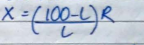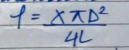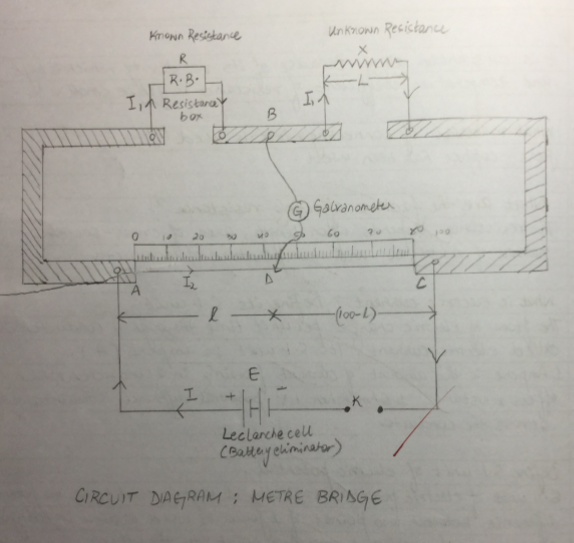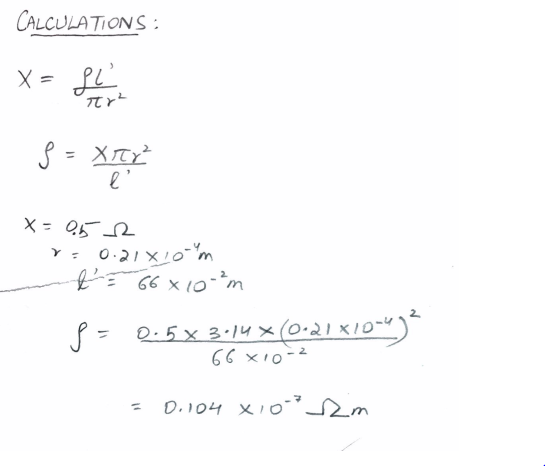# Finding the resistance of a given wire using meter bridge and hence determine resistivity of its material

Aim – To find the resistance of a given wire using meter bridge and hence determine resistivity of its material .

Apparatus

A meter bridge (slide wire bridge ) , a lecranche cell, a galvanometer , a resistor , a jockey , a one way key , a resistance wire , a screw gauge , a meter scale , a set of square , connecting wire , a piece of sand paper .

Theory :

The unknown resistance ‘X” is given by1. Where ‘R” is the known resistance placed in the left gap & unknown resistance ‘X” is the right gap of meter bridge .’L’ is length of meter bridge wire from zero end upto balance .
2. Resistivity of the material of the given wire is given byWhere ‘L’ is the length & D is the diameter of the given wire .

Procedure

1. Arrange the apparatus as shown in the arrangement diagram .
2. Connect the resistance wire whose resistance is to be determine in the right gap b/w C& B .Take care that no point /part of the wire forms a loop.
3. Connect resistance box of low range in the left gap b/w A & B .
4. Make all other connection as shown in the circuit diagram .
5. Take out some resistance from the resistance box , ping the key ‘K’
6. Touch the jockey gently first at length end & then right end of the bridge wire .
7. Note the deflection in the galvanometer. If the galvanometer shows deflection in the galvanometer reading in opposite direction the correction are correct. If the deflection is on one side only then there is fault in the circuit . Check & rectify the fault .
8. Move the jockey gently along the wire from left to right till gives zero deflection . The point where the jockey is touching the wire is null point ‘D’.
9. Choose an appropriate value of ‘R’ from the box such that there id no defection in the galvanometer when the jockey is nearly in the middle of the wire .
10. Note position of point ‘D’ to known the length AD=l
11. Take atleast 4 sets of observation in the same way by changing the value of R in the steps .
13. For specific resistance
1. Cut the resistance wire at the point where it leaves the terminal , started it & find its length by using a meter scale .
2. Measure the diameter of the wire at least at 4 places in two mutually perpendicular direction at each place with the of screw gauge .
3. Record your observation as given in the table .

Observation

1. Length of a given wire L = 66 cm =0.66 m
2. Table for unknown resistance (X)
 Resistance from box, R (Ohm) Length AB =l (cm) Length BC = (100-l) (cm) Unkown Resistance X = [R(100-l)]/L (ohm) 0.5 58.3 41.7 0.35 0.7 60.7 39.3 0.45 1 61.9 38.1 0.61 1.5 61.1 38.9 0.95 Mean = 0.59

3. Least count of screw gauge

Pitch of screw gauge =0.01

Total no of division on the circular scale =

LC of screw gauge = Pitch /No of the circular scale

Zero error (e) =(0)

Zero connection =(e)=0

 Main Scale Reading (mm) Circular Scale Reading Total Reading (diameter) (mm) Mean D (mm) Mean radius (D/2) (mm) 0 43 0.43 0.42 0.21 0 41 0.41

Result

1. The value of the unknown resistance X =0.5 ohm
2. The specific resistance of material of wire = 0.104 x10-3 ohm m
3. Percentage error

Precaution

The connection should be neat, clean & tight.

Source of error

Plug may not be clean

The wire may not be of uniform thickness.

Viva questions

1. Why is the meter bridge so called?

Since the bridge uses one meter long wire, it is called a meter bridge .

1. What is a null point ?

It is a point on the wire , keeping jockey at which galvanometer gives 0 deflection .

1. Why is the bridge method better that ohms law for measurement ?

It is so because the bridge method is a null method (at null point , no current is flowing in galvanometer ) and more sensitive .

1. Why copper strips used to press ends of wire are thick ?

Thick Cu strips have negligible resistance over the resistance of alloy meter bridge wire and minimize affect of end resistance .

Circuit diagramYou can also get Class XII Practicals on BiologyPhysics, and Physical Education.

## 23 Replies to “Finding the resistance of a given wire using meter bridge and hence determine resistivity of its material”

1.sushant says:

hiii
sir i am sushant raj from cbse i want to help in to my gmail physics pratical ..

2.trisha singh says:

Hii myself trisha singh of st thomas school,ranchi of isc board heartly grateful to u for ur best notes of exp related to phy practical.

1.Vishal Gupta says:

Accha

2.Vishal says:

Hi

3.Shivam singh says:

This is very good notes for intermediate students

4.gautam singh says:

standard value of the specific resistance of the material of the given. what?

5.Kritiman says:

Thanks guru

6.Amanjot singh says:

So gud notes sir its is so helpful to us

7.Muneeb shabir says:

8.Muneeb shabir says:

9.Vani says:

What is the material of wire used?? Please specify…..

10.md. samiul islam says:

meter bridge experiment discussion ???

11.Rebecca says:

The wire should have been of constantun as most of the time it is. But the result doesn’t match. Probably it is because of the experimental errors. It can be possible that he has taken a wire of different material. No need to worry as these problems would vanish once you perform the experiment yourself.

12.Hera alam says:

I want to know that when ‘ R’ is in left gap and when R is in right gap

13.Nikhil tyagi says:

gjb notes good carryon

14.Devyani jain says:

Thankuu so much. It helped me a lot for my practical experiment..I also recommended this site to my friends.. Wil you plz put up some questions of physics Sec -B expermt Nd full experiment also .

15.Savithri says:

Why 4 is not used in the final formula?

16.Saran says:

It is not 0.21 ×10^-4 m
It is 0.21×10^-3 m

17.Vaishnavi says:

***** 5 star

18.Ibrahim Nurudeen says:

But sir, what about the conclusion for the experiment

19.Ajay mahato says:

Awsome guru thanks for uploading this data it was really healful to me

20.Ayeni john says:

good day sir pls I need an answer to this:a battery of 1.50v has a terminal p.d of 1.25v when a resistor of 25ohms is joined to it.calculate the current flowing,the internal resistance r and the terminal p.d when a resistor of 10 ohms replaces the 25 ohms resistor. Thanks

21.Omkar Rathod says:

Specific resistance obesarvation